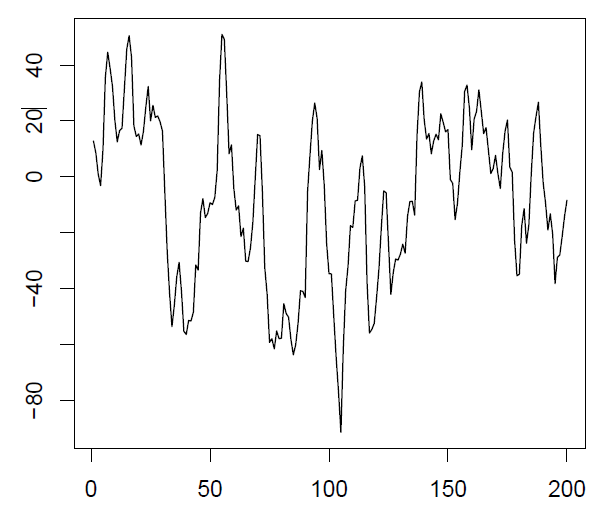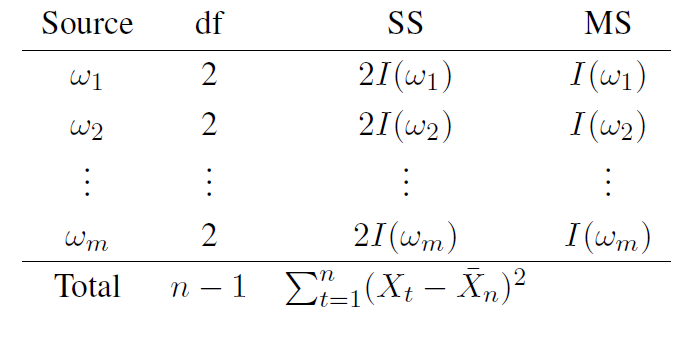# 4.2: The Spectral Density and the Periodogram

$$\newcommand{\vecs}{\overset { \rightharpoonup} {\mathbf{#1}} }$$ $$\newcommand{\vecd}{\overset{-\!-\!\rightharpoonup}{\vphantom{a}\smash {#1}}}$$$$\newcommand{\id}{\mathrm{id}}$$ $$\newcommand{\Span}{\mathrm{span}}$$ $$\newcommand{\kernel}{\mathrm{null}\,}$$ $$\newcommand{\range}{\mathrm{range}\,}$$ $$\newcommand{\RealPart}{\mathrm{Re}}$$ $$\newcommand{\ImaginaryPart}{\mathrm{Im}}$$ $$\newcommand{\Argument}{\mathrm{Arg}}$$ $$\newcommand{\norm}{\| #1 \|}$$ $$\newcommand{\inner}{\langle #1, #2 \rangle}$$ $$\newcommand{\Span}{\mathrm{span}}$$ $$\newcommand{\id}{\mathrm{id}}$$ $$\newcommand{\Span}{\mathrm{span}}$$ $$\newcommand{\kernel}{\mathrm{null}\,}$$ $$\newcommand{\range}{\mathrm{range}\,}$$ $$\newcommand{\RealPart}{\mathrm{Re}}$$ $$\newcommand{\ImaginaryPart}{\mathrm{Im}}$$ $$\newcommand{\Argument}{\mathrm{Arg}}$$ $$\newcommand{\norm}{\| #1 \|}$$ $$\newcommand{\inner}{\langle #1, #2 \rangle}$$ $$\newcommand{\Span}{\mathrm{span}}$$$$\newcommand{\AA}{\unicode[.8,0]{x212B}}$$

The fundamental technical result which is at the core of spectral analysis states that any (weakly) stationary time series can be viewed (approximately) as a random superposition of sine and cosine functions varying at various frequencies. In other words, the regression in (4.1.1) is approximately true for all weakly stationary time series. In Chapters 1-3, it is shown how the characteristics of a stationary stochastic process can be described in terms of its ACVF $$\gamma(h)$$. The first goal in this section is to introduce the quantity corresponding to $$\gamma(h)$$ in the frequency domain.

Definition 4.2.1 (Spectral Density)

If the ACVF $$\gamma(h)$$ of a stationary time series (Xt)t\in\mathbb{Z} \nonumber \] satisfies the condition

$\sum_{h=-\infty}^\infty|\gamma(h)|<\infty, \nonumber$

then there exists a function f defined on (-1/2,1/2] such that

$\gamma(h)=\int_{-1/2}^{1/2}\exp(2\pi i\omega h)f(\omega)d\omega,\qquad h\in\mathbb{Z}, \nonumber$

and

$f(\omega)=\sum_{h=-\infty}^\infty\gamma(h)\exp(-2\pi i\omega h),\qquad\omega\in(-1/2,1/2]. \nonumber$

The function f is called the spectral density of the process $$X_t\colon t\in\mathbb{Z})$$.

Definition 4.2.1 (which contains a theorem part as well) establishes that each weakly stationary process can be equivalently described in terms of its ACVF or its spectral density. It also provides the formulas to compute one from the other. Time series analysis can consequently be performed either in the time domain (using $$\gamma(h)$$) or in the frequency domain (using f$$(\omega))$$. Which approach is the more suitable one cannot be decided in a general fashion but has to be reevaluated for every application of interest.

In the following, several basic properties of the spectral density are collected and evaluated f for several important examples. That the spectral density is analogous to a probability density function is established in the next proposition.

Proposition 4.2.1

If f($$\omega$$) is the spectral density of a weakly stationary process $$(X_t\colon t\in\mathbb{Z})$$, then the following statements hold:

1. f($$\omega$$) $$\geq$$ 0 for all $$\omega$$. This follows from the positive definiteness of $$\gamma(h)$$
2. f($$\omega$$)=f(-$$\omega$$) and f($$\omega+1$$)=f($$\omega$$)
3. The variance of ($$X_t\colon t\in\mathbb{Z})$$ is given by

$\gamma(0)=\int_{-1/2}^{1/2}f(\omega)d\omega. \nonumber$

Part (c) of the proposition states that the variance of a weakly stationary process is equal to the integrated spectral density over all frequencies. This property is revisited below, when a spectral analysis of variance (spectral ANOVA) will be discussed. In the following three examples are presented.

Example 4.2.1 (White Noise)

If $$(Z_t\colon t\in\mathbb{Z})\sim\mbox{WN}(0,\sigma^2)$$, then its ACVF is nonzero only for h=0, in which case $$\gamma_Z(h)=\sigma^2$$. Plugging this result into the defining equation in Definition4.2.1 yields that

$f_Z(\omega)=\gamma_Z(0)\exp(-2\pi i\omega 0)=\sigma^2. \nonumber$

The spectral density of a white noise sequence is therefore constant for all $$\omega\in(-1/2,1/2]$$, which means that every frequency $$\omega$$ contributes equally to the overall spectrum. This explains the term white'' noise (in analogy to white'' light).

Example 4.2.2 (Moving Average)

Let $$(Z_t\colon t\in\mathbb{Z})\sim\mbox{WN}(0,\sigma^2)$$ and define the time series $$(X_t\colon t\in\mathbb{Z})$$ by

$X_t=\tfrac 12\left(Z_t+Z_{t-1}\right),\qquad t\in\mathbb{Z}. \nonumber$

It can be shown that

$\gamma_X(h)=\frac{\sigma^2}4\left(2-|h|\right),\qquad h=0,\pm 1 \nonumber$Figure 4.3: Time series plot of white noise $$(Z_t\colon t\in\mathbb{Z})$$ (left), two-point moving average $$(X_t\colon t\in\mathbb{Z})$$ (middle) and spectral density of $$(X_t\colon t\in\mathbb{Z})$$ (right).

and that $$\gamma$$_X=0 otherwise. Therefore,

$f_X(\omega)=\sum_{h=-1}^1\gamma_X(h)\exp(2\pi i \omega h) \nonumber$

$= \frac{\sigma^2}4 (\exp(-2\pi i \omega (-1)))+2\exp(-2\pi i \omega 0)+\exp(-2\pi i \omega 1) \nonumber$

$= \frac{\sigma^2}2 (1+\cos(2\pi\omega)) \nonumber$

using that $$\exp(ix)=\cos(x)+i\sin(x)$$, $$\cos(x)=\cos(-x)$$ and $$\sin(x)=-\sin(-x)$$. It can be seen from the two time series plots in Figure 4.3 that the application of the two-point moving average to the white noise sequence smoothes the sample path. This is due to an attenuation of the higher frequencies which is visible in the form of the spectral density in the right panel of Figure 4.3. All plots have been obtained using Gaussian white noise with $$\sigma^2=1$$.

Example 4.2.3 (AR(2) Process).

Let $$(X_t\colon t\in\mathbb{Z})$$ be an AR(2) process which can be written in the form

$Z_t=X_t-\phi_1X_{t-1}-\phi_2X_{t-2},\qquad t\in\mathbb{Z} \nonumber$

In this representation, it can be seen that the ACVF $$\gamma_Z$$ of the white noise sequence can be obtained as

$\gamma_Z(h) = E [(X_t-\phi_1X_{t-1}-\phi_2X_{t-2}) (X_{t+h}-\phi_1X_{t+h-1}-\phi_2X_{t+h-2})] \nonumber$

$=(1+\phi_1^2+\phi_2^2)\gamma_X(h)+(\phi_1\phi_2-\phi_1)[\gamma_X(h+1)+\gamma_X(h-1)] \nonumber$

$\qquad - \phi_2[\gamma_X(h+2)+\gamma_X(h-2)] \nonumber$

Now it is known from Definition 4.2.1 that

$\gamma_X(h)=\int_{-1/2}^{1/2}\exp(2\pi i\omega h)f_X(\omega)d\omega \nonumber$

and

$\gamma_Z(h)=\int_{-1/2}^{1/2}\exp(2\pi i\omega h)f_Z(\omega)d\omega, \nonumber$Figure 4.4: Time series plot and spectral density of the AR(2) process in Example 4.2.3.

where $$f_X(\omega)$$ and $$f_Z(\omega)$$ denote the respective spectral densities. Consequently,

$\gamma_Z(h)=\int_{-1/2}^{1/2}\exp(2\pi i\omega h)f_Z(\omega)d\omega \\[.2cm] \nonumber$

$=(1+\phi_1^2+\phi_2^2)\gamma_X(h)+(\phi_1\phi_2-\phi_1)[\gamma_X(h+1)+\gamma_X(h-1)]-\phi_2[\gamma_X(h+2)+\gamma_X(h-2)]\\[.2cm] \nonumber$

$=\int_{-1/2}^{1/2}\left[(1+\phi_1^2+\phi_2^2)+(\phi_1\phi_2-\phi_1)(\exp(2\pi i\omega)+\exp(-2\pi i\omega))\right. \\[.2cm] \nonumber$

$\qquad\qquad\left. -\phi_2(\exp(4\pi i \omega)+\exp(-4\pi i \omega)) \right]\exp(2\pi i\omega h)f_X(\omega)d\omega \\[.2cm] \nonumber$

$=\int_{-1/2}^{1/2}\left[(1+\phi_1^2+\phi_2^2)+2(\phi_1\phi_2-\phi_1)\cos(2\pi\omega)-2\phi_2\cos(4\pi\omega)\right]\exp(2\pi i\omega h)f_X(\omega)d\omega. \nonumber$

The foregoing implies together with $$f_Z(\omega)=\sigma^2$$ that

$\sigma^2=\left[(1+\phi_1^2+\phi_2^2)+2(\phi_1\phi_2-\phi_1)\cos(2\pi\omega)-2\phi_2\cos(4\pi\omega)\right]f_X(\omega). \nonumber$

Hence, the spectral density of an AR(2) process has the form

$f_X(\omega)=\sigma^2\left[(1+\phi_1^2+\phi_2^2)+2(\phi_1\phi_2-\phi_1)\cos(2\pi\omega)-2\phi_2\cos(4\pi\omega)\right]^{-1}. \nonumber$

Figure 4.4 displays the time series plot of an AR(2) process with parameters $$\phi_1=1.35$$, $$\phi_2=-.41$$ and $$\sigma^2=89.34$$. These values are very similar to the ones obtained for the recruitment series in Section 3.5. The same figure also shows the corresponding spectral density using the formula just derived.

With the contents of this Section, it has so far been established that the spectral density $f(\omega)$ is a population quantity describing the impact of the various periodic components. Next, it is verified that the periodogram $I(\omega_j)$ introduced in Section \ref{sec:4.1} is the sample counterpart of the spectral density.

Proposition 4.2.2.

Let $$\omega_j=j/n$$ denote the Fourier frequencies. If $$I(\omega_j)=|d(\omega_j)|^2$$ is the periodogram based on observations $$X_1,\ldots,X_n$$ of a weakly stationary process $$(X_t\colon t\in\mathbb{Z})$$, then

$I(\omega_j)=\sum_{h=-n+1}^{n-1}\hat{\gamma}_n(h)\exp(-2\pi i \omega_j h),\qquad j\not=0. \nonumber$

If $$j=0$$, then $$I(\omega_0)=I(0)=n\bar X_n^2$$.

Proof. Let first $$j\not= 0$$. Using that $$\sum_{t=1}^n\exp(-2\pi i\omega_jt)=0$$, it follows that

$I(\omega_j) = \frac 1n\sum_{t=1}^n\sum_{s=1}^n(X_t-\bar X_n)(X_s-\bar X_n)\exp(-2\pi i\omega_j(t-s))\\[.2cm] \nonumber$

$=\frac 1n \sum_{h=-n+1}^{n-1}\sum_{t=1}^{n-|h|}(X_{t+|h|}-\bar X_n)(X_t-\bar X_n)\exp(-2\pi i\omega_jh)\\[.2cm] \nonumber$

$=\sum_{h=-n+1}^{n-1}\hat\gamma_n(h)\exp(-2\pi i\omega_jh), \nonumber$

which proves the first claim of the proposition. If $$j=0$$, the relations $$\cos(0)=1$$ and $$\sin(0)=0$$ imply that $$I(0)=n\bar X_n^2$$. This completes the proof.

More can be said about the periodogram. In fact, one can interpret spectral analysis as a spectral analysis of variance (ANOVA). To see this, let first

$d_c(\omega_j) = \mathrm{Re}(d(\omega_j))=\frac{1}{\sqrt{n}}\sum_{t=1}^nX_t\cos(2\pi\omega_jt), \\[.2cm] \nonumber$

$d_s(\omega_j) = \mathrm{Im}(d(\omega_j))=\frac{1}{\sqrt{n}}\sum_{t=1}^nX_t\sin(2\pi\omega_jt). \nonumber$

Then, $$I(\omega_j)=d_c^2(\omega_j)+d_s^2(\omega_j)$$. Let us now go back to the introductory example and study the process

$X_t=A_0+\sum_{j=1}^m\big[A_j\cos(2\pi\omega_j t)+B_j\sin(2\pi\omega_jt)\big], \nonumber$

where $$m=(n-1)/2$$ and $$n$$ odd. Suppose $$X_1,\ldots,X_n$$ have been observed. Then, using regression techniques as before, it can be seen that $$A_0=\bar{X}_n$$ and

$A_j = \frac 2n\sum_{t=1}^nX_t\cos(2\pi\omega_jt)=\frac{2}{\sqrt{n}}d_c(\omega_j),\\[.2cm] \nonumber$

$B_j = \frac 2n\sum_{t=1}^nX_t\sin(2\pi\omega_jt)=\frac{2}{\sqrt{n}}d_s(\omega_j). \nonumber$

Therefore,

$\sum_{t=1}^n(X_t-\bar{X}_n)^2=2\sum_{j=1}^m\big[d_c^2(\omega_j)+d_s^2(\omega_j)\big]=2\sum_{j=1}^mI(\omega_j) \nonumber$

and the following ANOVA table is obtained. If the underlying stochastic process exhibits a strong periodic pattern at a certain frequency, then the periodogram will most likely pick these up.Example 4.2.4

Consider the $$n=5$$ data points $$X_1=2$$, $$X_2=4$$, $$X_3=6$$, $$X_4=4$$ and $$X_5=2$$, which display a cyclical but nonsinusoidal pattern. This suggests that $$\omega=1/5$$ is significant and $$\omega=2/5$$ is not. In R, the spectral ANOVA can be produced as follows.

>x = c(2,4,6,4,2), t=1:5

>cos1 = cos(2*pi*t*1/5)

>sin1 = sin(2*pi*t*1/5)

>cos2 = cos(2*pi*t*2/5)

>sin2 = sin(2*pi*t*2/5)

This generates the data and the independent cosine and sine variables. Now run a regression and check the ANOVA output.

>reg = lm(x\~{}cos1+sin1+cos2+sin2)

>anova(reg)

This leads to the following output.

Response: x

Df Sum Sq Mean Sq F value Pr(>F)

cos1 1 7.1777 7.1777

cos2 1 0.0223 0.0223

sin1 1 3.7889 3.7889

sin2 1 0.2111 0.2111

Residuals 0 0.0000

According to previous reasoning (check the last table!), the periodogram at frequency $$\omega_1=1/5$$ is given as the sum of the $$cos1$$ and $$\tt sin1$$ coefficients, that is, $$I(1/5)=(d_c(1/5)+d_s(1/5))/2=(7.1777+3.7889)/2=5.4833$$. Similarly, $$I(2/5)=(d_c(2/5)+d_s(2/5))/2=(0.0223+0.2111)/2=0.1167.$$

Note, however, that the mean squared error is computed differently in R. We can compare these values with the periodogram:

> abs(fft(x))$$\widehat{}$$ 2/5

 64.8000000 5.4832816 0.1167184 0.1167184 5.4832816

The first value here is $$I(0)=n\bar{X}_n^2=5*(18/5)^2=64.8$$. The second and third value are $$I(1/5)$$ and $$I(2/5)$$, respectively, while $$I(3/5)=I(2/5)$$ and $$I(4/5)=I(1/5)$$ complete the list.

In the next section, some large sample properties of the periodogram are discussed to get a better understanding of spectral analysis. \

## Contributers

This page titled 4.2: The Spectral Density and the Periodogram is shared under a not declared license and was authored, remixed, and/or curated by Alexander Aue.